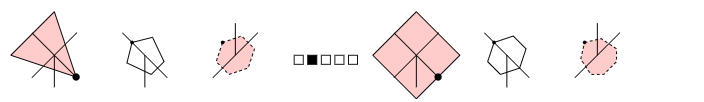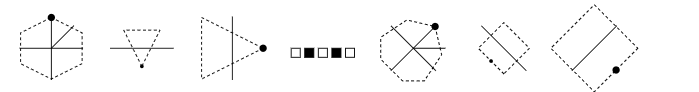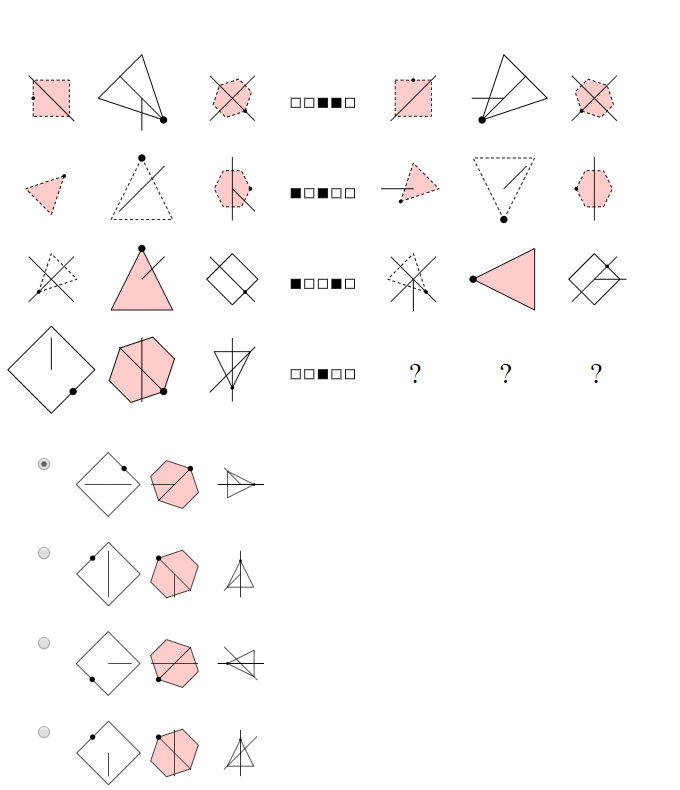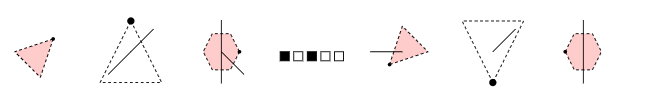# Abstract Reasoning ExplanationAbstract reasoning tests how well you can identify relationships and patterns in a series of objects. Abstract reasoning is mainly about gaining insight, so we will minimize the explanation here and work out two examples.

Example 1

Which figures should be on the question marks?In this type of exercise, you have the starting figures on the left, a transformation is applied to that and then you get the end figures on the right. The middle section consists of five buttons that can be set to "on" (black) or "off" (white).

The assignment is to see what happens to the bottom three starting figures when buttons 3 and 4 are “on”. For this we need to figure out what effect button 3 and button 4 have on the starting figures. Buttons 3 and 4 are active in the second and third rows. It is often easy to start with a row where only 1 button is "on". In this case, this is the second row.You can see that the figures on the right have a dotted line while the figures on the left have a normal line and vice versa where the figures on the left have a dotted line already. This means that the third button transforms a normal line into a dotted line and a dotted line into a normal line.

We now have to find out what the effect of the fourth button is. The fourth button is "on" in the third row, but it also has button 2 on. In the first row, we can see what button 1 does, so we look at it first.You see that a triangle changes to a quadrangle, a pentagon to a hexagon and a hexagon to a heptagon. This means that button 2 adds an angle to the figures.

Now we can look at the third row to see what effect button 4 has.You can see that through button 2 all figures on the right have an angle more than the figures on the left. In addition, all figures are rotated 45 degrees clockwise.

To find which figures should be on the question marks, we must, therefore, apply the following transformation to the figures on the left:

• Normal lines become dotted lines and vice versa
• All figures must be rotated 45 degrees clockwise.

You see that the answer must be D (the bottom option).